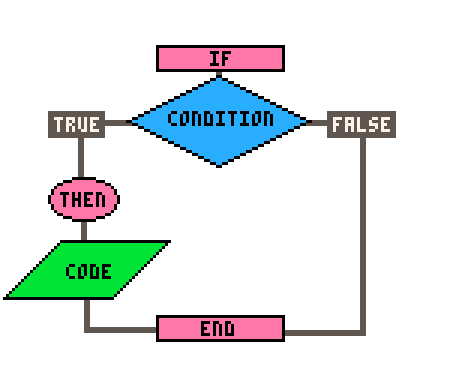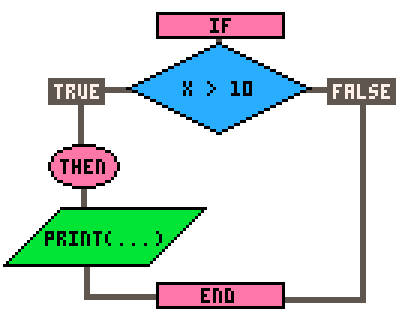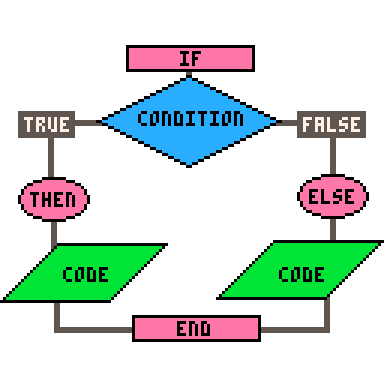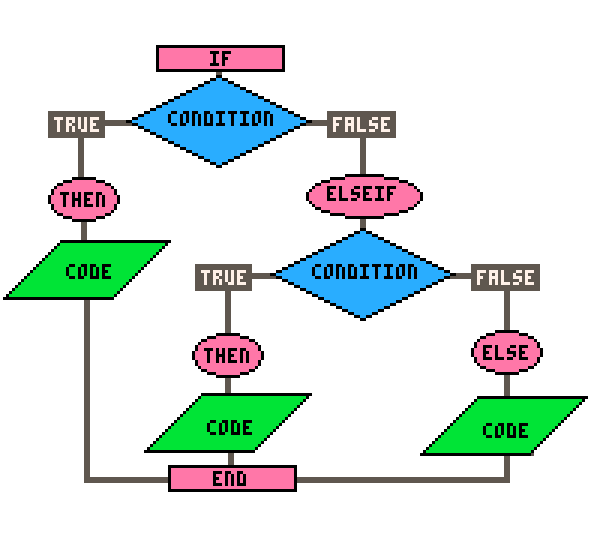# IF

The word "condition" means a mode or state of being.
a light can be on or off; you can be happy or sad or mad

The word "conditional" means depending on a mode or state of being.
if the light is off then turn it on; if you are happy then I am happy

In programming, conditionals are exactly like this, but there are only two options: true or false. Writing conditionals is like playing the game 21 Questions with your computer. Just like that game, you can only ask Yes or No questions. However, instead of yes and no, the computer will respond with either `True` or `False`.

## If

Conditionals are used to execute different blocks of code depending on whether a condition is true or false. In other words, conditionals allow you to ask a true or false question, then run certain code only if that is true. We can draw a conditional like this in a flowchart:In code, this simple conditional is written:

``````if condition then
--code
end
``````

Here's an actual example where we want to know if a variable named `x` is greater than `10`:

``````if x > 10 then
print("x is greater than 10")
end``````In the above example, the "if" statement checks whether the variable "`x`" is greater than 10. If it is, the code inside the block ( in this case, the "`print()`" statement ) will be executed. If the condition is not true, the code inside the block will be skipped and the conditional is closed with `end`.## If Else

You can also use an "`if else`" statement to execute different blocks of code depending on whether a condition is true or false.Here's an example:

``````if x > 10 then
print("x is greater than 10")
else
print("x is less than or equal to 10")
end``````

In the above example, if "`x`" is greater than 10, the code inside the first block will be executed. If "`x`" is less than or equal to 10, the code inside the second block will be executed.## If Elseif Else

Finally, you can use an "`if elseif else`" statement to test multiple conditions.Here's an example:

``````if x > 10 then
print("x is greater than 10")
elseif x == 10 then
print("x is equal to 10")
else
print("x is less than 10")
end``````

In the above example, if "`x`" is greater than 10, the code inside the first block will be executed. If "`x`" is equal to 10, the code inside the second block will be executed. If neither condition is true, the code inside the third block will be executed.

You can use as many `elseif` conditionals as you'd like, but keep in mind that long if/elseif statements like that can use a lot of processing power to check every condition before getting to the last one, especially if you run these checks every frame of the game.Here are some tips to keep in mind to make these long conditional statements more readable and efficient:

2. Put the most likely conditions first: This will help evaluate the condition faster and reduce the number of unnecessary condition checks.

3. Limit the number of conditions: If you have too many conditions, consider refactoring your code to use a different approach, such as a loop or a table lookup.

4. Use else to catch all other cases: This will help you avoid unexpected behavior if your conditions do not cover all possible cases.

5. Organize your code into tables or functions: If your if-elseif statements are getting too long, consider breaking up your code into smaller functions. This will help you better organize your code and make it more maintainable.Take note of these things:

`if` is the opening of the statement and requires three things: a condition, followed by a `then`, and closed with an `end`.

- `if` conditions may have parentheses but do not require them, unless using the shorthand form (more below).

- `elseif` is a single word without spaces.

- `elseif` requires a condition followed by a `then` but does not have an `end` for itself.

- `else` does not have a condition, `then`, nor `end` for itself.#### Not for Beginners

Shorthand `if`statement:

``if (condition) code``

Parentheses are required for this, it must be on one line, and must not have `then`, `elseif`, `else`, nor `end`. Here is an example:

``if (x>10) print("x is greater than 10")``

A common usage for this is when you have multiple simple if statements such as checking for button presses. For example:

``````--longform
if btn(0) then x-=1 end
if btn(1) then x+=1 end
if btn(2) then y-=1 end
if btn(3) then y+=1 end

--shorthand
if (btn(0)) x-=1
if (btn(1)) x+=1
if (btn(2)) y-=1
if (btn(3)) y+=1``````

#### (Note: This shorthand does not cost less tokens.)

Shorthand Assignments using `if elseif else` ("ternary operator" in other languages):

``x = condition and a or b``

This will check if the condition is true, then set `X` to `A`, but if the condition is false, then set `X` to `B`.

Warning: This breaks if `A` is `false` or `nil`.

An example of this, while checking for button inputs, could be:

``````--longform
if btn(0) then
player_dx = -1
else
player_dx = 0
end

--shorthand if else
player_dx = btn(0) and -1 or 0
``````

This shorthand form will set a player_dx variable to negaitve one if the player presses button left (#0) or to zero if not pressing button left.

You can even add an elseif secondary check using another pair of `and or` conditionals.

``````--longform
if btn(0) then
player_dx = -1
elseif btn(1) then
player_dx = 1
else
player_dx = 0
end

--shorthand if elseif else
player_dx = btn(0) and -1 or btn(1) and 1 or 0
``````

This shorthand will check if button left (#0) is pressed, then set player_dx to negative one but if button left is false, then it will also check if button right is pressed, then set player_dx to one, but if both are false, then set player_dx to zero.469

8 Sep 2023

`Font`